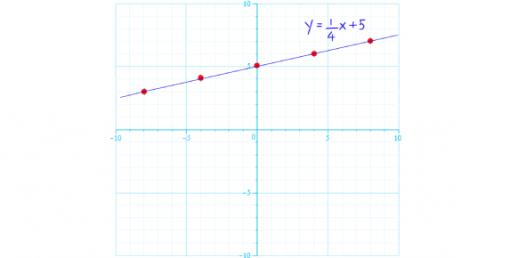# Math: System Of Linear Equations! Quiz

10 Questions | Total Attempts: 680SettingsDo you know how to solve a linear equation problem? There is a language to math that needs to be learned to understand it. In mathematics, a mathematical expression is a grouping of symbols that are well informed according to the rules of the context. It is a combination of connections based upon the signs: subtraction, addition, multiplication, and division. Take this fun quiz to test your math IQ.

• 1.
Which line through the given points is steeper. Show how you got the answer. Line 1: (-2,3)(3,5) Line 2: (3,1)(6,5)
• A.

Line 1

• B.

Line 2

• 2.
Tell whether the lines through the given points are parallel, perpendicular or neither. Line 1: (1,0)(7,4) Line 2: (7,0)(3,6)
• A.

Parallel

• B.

Perpendicular

• C.

Neither

• 3.
Find the slope of the line that passes through the following points:(3,5  )   (5,6)
• A.

1/4

• B.

1/2

• C.

-1/4

• D.

-1/2

• 4.
Write the equation  of a line given point P (0,5) AND HAS A SLOPE OF 2/3
• A.

Y= 3/2 x +5

• B.

Y= 2/3 x

• C.

Y= 2/3 X +5

• D.

Y= 2/3 x -5

• 5.
Which lines are parallel?y=3x-4x+3y=63(x+1)=y-2
• A.

1st and 2nd ones

• B.

2nd and 3rd ones

• C.

1st and 3rd ones

• D.

All three are parallel to each other

• E.

None are parallel to each other

• 6.
Which lines are parallel?x-6y=106x-y=11x+6y=12
• A.

1st and 2nd ones

• B.

2nd and 3rd ones

• C.

1st and 3rd ones

• D.

All three are parallel to each other

• E.

None are parallel to each other

• 7.
Write an equation that passes through point (-1,0) and has a slope of -1
• A.

Y=x+1

• B.

Y=-x-1

• C.

Y=2x-1

• D.

Y=-2x+1

• 8.
Write an equation that passes through point (5,4) and has a slope of 4
• A.

Y= -4x-16

• B.

Y=4x+16

• C.

Y=2x-16

• D.

Y=4x-16

• 9.
Write an equation of a line with a slope of -5 and a y-intercept of -12
• A.

Y= 5x-12

• B.

Y= -5x-12

• C.

Y= -5x+12

• D.

Y= -12

• 10.
Write an equation of a line with a slope of 3 and a y-intercept of 2
• A.

Y= 2x +3

• B.

Y= -2x +3

• C.

Y=3x+2

• D.

Y= 2x -3

Related TopicsBack to top